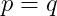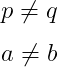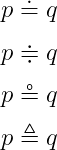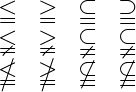# How to write a equal(=) or not equal(≠) symbol in LaTeX?

Mathematically, the use of equals and not equal symbols is much more. Equal symbols are represented by attaching two horizontal bars.

 Properties Value Symbol Equal Package Default Command = Argument No Example =

You can easily identify equal symbols. Because Equal Symbol has this on your keyboard.

\documentclass{article}
\begin{document}
$p = q$
\end{document}

Output :So, notice this program above, the direct symbol is used here.

However, you can use both \neq and \ne commands in the case of not equal symbols.

\documentclass{article}
\begin{document}
$p \neq q$
$a \ne b$
\end{document}

Output :Also, you have noticed that dot, triangle, and circle are used on equal symbol in different cases.

\documentclass{article}
\usepackage{amssymb}
\begin{document}
$p \doteq q$
$p \doteqdot q$
$p \circeq q$
$p \triangleq q$
\end{document}

Output :And greater than, less than, subset, and super subset with equal and not equal symbols used.

\documentclass{article}
\usepackage{amsmath,amssymb}
\begin{document}
$\begin{matrix} \leqq & \geqq & \subseteqq & \supseteqq \\ \lneqq & \gneqq & \subsetneqq & \subsetneqq \\ \nleqq & \ngeqq & \nsubseteqq & \nsubseteqq \end{matrix}$
\end{document}

Output :#### Md Jidan Mondal

LaTeX expert with over 10 years of experience in document preparation and typesetting. Specializes in creating professional documents, reports, and presentations using LaTeX.